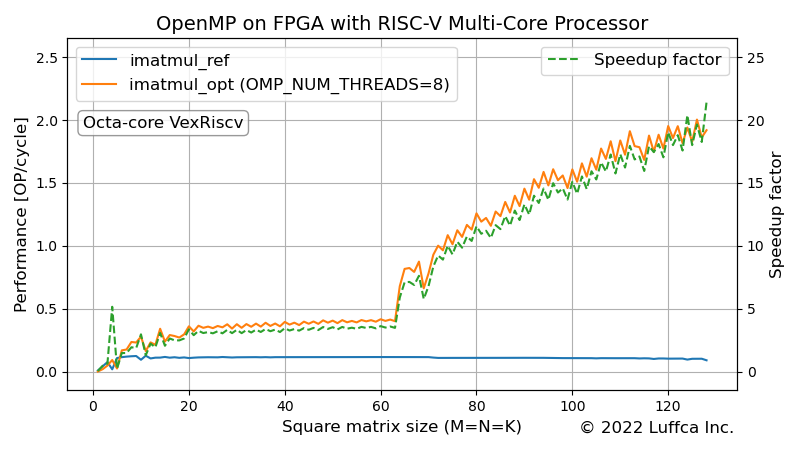# OpenMP on FPGA with RISC-V Multi-Core ProcessorWe have implemented a RISC-V multi-core processor on an FPGA board and evaluated the performance of the matrix multiplication kernel using OpenMP.

See related articles here.

## OpenMP

Wikipedia describes OpenMP as follows:

OpenMP (Open Multi-Processing) is an application programming interface (API) that supports multi-platform shared-memory multiprocessing programming in C, C++, and Fortran, on many platforms, instruction-set architectures and operating systems, including Solaris, AIX, FreeBSD, HP-UX, Linux, macOS, and Windows.

## Matrix Multiplication

The related articles Matrix Multiplication based on the RISC-V Vector Extension and Tiny Matrix Extension using RISC-V Custom Instructions accelerated the matrix multiplication kernel using the RISC-V Vector Extension and RISC-V Custom Instructions respectively. In this article, we use OpenMP to speed up the matrix multiplication kernel.

The matrix multiplication kernel computes the M × N matrix C, which is the product of the M × K matrix A and the K × N matrix B. The code for the reference kernel `imatmul_ref` is as follows.

```// C = AB with A = [M x K], B = [K x N], C = [M x N]
void imatmul_ref(const int M, const int N, const int K, const int8_t* A,
const int8_t* B, int32_t* C) {
int i, j, k;
int32_t sum;
for (i = 0; i < M; ++i) {
for (j = 0; j < N; ++j) {
sum = 0;
for (k = 0; k < K; ++k) {
sum += A[i * K + k] * B[k * N + j];
}
C[i * N + j] = sum;
}
}
}
```

## RISC-V Multi-core System

For the performance evaluation of the matrix multiplication kernel using OpenMP, we used Digilent’s FPGA board Nexys Video, which implements the RISC-V multi-core system shown below.

• Processor: Octa-core VexRiscv
• ISA: RV32IMAFDC (RV32GC)
• Operating frequency: 100 MHz
• DRAM: 512 MiB
• OS: Linux

## Matrix Multiplication using OpenMP on RISC-V Multi-core

The featured image shows the performance of the matrix multiplication kernel `imatmul_opt` using OpenMP. Performance [OP/cycle] is calculated using the average of 10 program executions.

If the square matrix size (M=N=K) is less than 64, the kernel is running in single thread, because the parallelization overhead is large and the parallelization effect is low for small matrices.

For square matrix sizes of 32, 64, and 128, we achieved speedups of 3.30, 5.89, and 21.42 times, respectively, compared to the reference kernel.

## Summary

We have implemented a RISC-V multi-core processor, octa-core VexRiscv, on an FPGA board and evaluated the performance of the matrix multiplication kernel using OpenMP.

Previous article

Next article